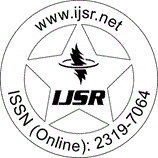International Journal of Science and Research (IJSR)
Call for Papers | Fully Refereed | Open Access | Double Blind Peer Reviewed

Research Paper | Mathematics | India | Volume 5 Issue 8, August 2016

# Some New Results on Absolute Difference of Cubic and Square Sum Labeling of a Class of Graphs

Dr. Mathew Varkey T K | Sunoj B S

Abstract: A graph labeling is an assignment of integers to the vertices or edges or both subject to certain conditions. In this paper, we introduce the new concept, an absolute difference of cubic and square sum labeling of a graph. The graph for which every edge label is the absolute difference of the sum of the cubes of the end vertices and the sum of the squares of the end vertices. It is also observed that the weights of the edges are found to be multiples of 2. Here we characterize few graphs for cubic and square sum labeling.

Keywords: Graph labeling, cycle graph, fan graph square sum graphs, cubic graphs

Edition: Volume 5 Issue 8, August 2016,

Pages: 1465 - 1467